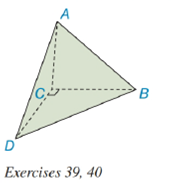Chapter 9.2, Problem 40E### Elementary Geometry for College St...

6th Edition
Daniel C. Alexander + 1 other
ISBN: 9781285195698

#### Solutions

Chapter
Section### Elementary Geometry for College St...

6th Edition
Daniel C. Alexander + 1 other
ISBN: 9781285195698
Textbook Problem
1 views

# Exercise 39 and 40 are based upon the “uniqueness of volume.”The volume of the pyramid shown is 32 i n 3 .a) Find the area of base △ A C D if the length of the altitude from vertex B to base △ A C D is 4 i n . b) To the nearest tenth of an inch, find the length of the altitude from vertex C to base △ A B D if A A B D ≈ 31.23 i n 2 .To determine

(a)

To find:

The area of base ACD.

Explanation

Given:

A tetrahedron (not rectangular) has vertices at A, B, C, and D. The volume of the pyramid shown is 32in3 as below,

and the length of the altitude from vertex B to base ACD is 4in.

Properties Used:

The volume V of a pyramid having a base area B and an altitude of length h is given by V=13Bh.

Area of a right-angled triangle is 12×base×height.

Approach Used:

i) Substitute the values of height h and the volume V in equation V=13Bh to find the value of area B.

Calculation:

From the given figure

To determine

(b)

To find:

The length of the altitude from vertex C to base ABD to the nearest tenth of an inch.

### Still sussing out bartleby?

Check out a sample textbook solution.

See a sample solution

#### The Solution to Your Study Problems

Bartleby provides explanations to thousands of textbook problems written by our experts, many with advanced degrees!

Get Started